# 大数据开发！Pandas转spark无痛指南！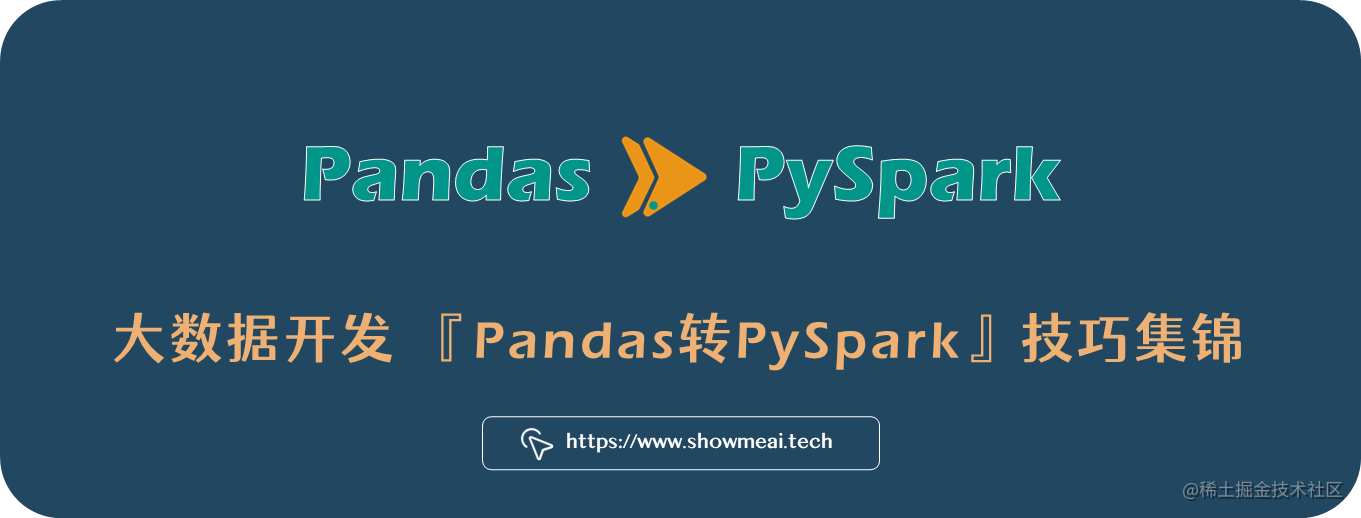Pandas 是每位数据科学家和 Python 数据分析师都熟悉的工具库，它灵活且强大具备丰富的功能，但在处理大型数据集时，它是非常受限的。# 💡 导入工具库

``````# pandas vs pyspark，工具库导入
import pandas as pd
import pyspark.sql.functions as F

PySpark 所有功能的入口点是 SparkSession 类。通过 SparkSession 实例，您可以创建spark dataframe、应用各种转换、读取和写入文件等，下面是定义 SparkSession的代码模板：

``````from pyspark.sql import SparkSession
spark = SparkSession\
.builder\
.appName('SparkByExamples.com')\
.getOrCreate()

# 💡 创建 dataframe

## 💦 Pandas

``````columns = ["employee","department","state","salary","age"]
data = [("Alain","Sales","Paris",60000,34),
("Ahmed","Sales","Lyon",80000,45),
("Ines","Sales","Nice",55000,30),
("Fatima","Finance","Paris",90000,28),
("Marie","Finance","Nantes",100000,40)]

``````df = pd.DataFrame(data=data, columns=columns)
# 查看头2行

## 💦 PySpark

``````df = spark.createDataFrame(data).toDF(*columns)
# 查看头2行
df.limit(2).show()

# 💡 指定列类型

## 💦 Pandas

Pandas 指定字段数据类型的方法如下：

``````types_dict = {
"employee": pd.Series([r for r in data], dtype='str'),
"department": pd.Series([r for r in data], dtype='str'),
"state": pd.Series([r for r in data], dtype='str'),
"salary": pd.Series([r for r in data], dtype='int'),
"age": pd.Series([r for r in data], dtype='int')
}

df = pd.DataFrame(types_dict)

Pandas 可以通过如下代码来检查数据类型：

``````df.dtypes

## 💦 PySpark

PySpark 指定字段数据类型的方法如下：

``````from pyspark.sql.types import StructType,StructField, StringType, IntegerType

schema = StructType([ \
StructField("employee",StringType(),True), \
StructField("department",StringType(),True), \
StructField("state",StringType(),True), \
StructField("salary", IntegerType(), True), \
StructField("age", IntegerType(), True) \
])

df = spark.createDataFrame(data=data,schema=schema)

PySpark 可以通过如下代码来检查数据类型：

``````df.dtypes
# 查看数据类型
df.printSchema()

# 💡 读写文件

Pandas 和 PySpark 中的读写文件方式非常相似。 具体语法对比如下：

## 💦 Pandas

``````df = pd.read_csv(path, sep=';', header=True)
df.to_csv(path, ';', index=False)

## 💦 PySpark

``````df = spark.read.csv(path, sep=';')
df.coalesce(n).write.mode('overwrite').csv(path, sep=';')

### 注意 ①

PySpark 中可以指定要分区的列：

``````df.partitionBy("department","state").write.mode('overwrite').csv(path, sep=';')

# 💡 数据选择 - 列

## 💦 Pandas

``````columns_subset = ['employee', 'salary']

## 💦 PySpark

``````columns_subset = ['employee', 'salary']

df.select(columns_subset).show(5)

# 💡 数据选择 - 行

## 💦 Pandas

Pandas可以使用 `iloc`对行进行筛选：

``````# 头2行

## 💦 PySpark

``````df.take(2).head()
# 或者

# 💡 条件选择

## 💦 Pandas

Pandas 中根据特定条件过滤数据/选择数据的语法如下：

``````# First method
flt = (df['salary'] >= 90_000) & (df['state'] == 'Paris')
filtered_df = df[flt]

# Second Method: Using query which is generally faster
filtered_df = df.query('(salary >= 90_000) and (state == "Paris")')
# Or
target_state = "Paris"
filtered_df = df.query('(salary >= 90_000) and (state == @target_state)')

## 💦 PySpark

``````# 方法1：基于filter进行数据选择
filtered_df = df.filter((F.col('salary') >= 90_000) & (F.col('state') == 'Paris'))

# 或者
filtered_df = df.filter(F.expr('(salary >= 90000) and (state == "Paris")'))

# 方法2：基于SQL进行数据选择
df.createOrReplaceTempView("people")

filtered_df = spark.sql("""
SELECT * FROM people
WHERE (salary >= 90000) and (state == "Paris")
""")

# 💡 添加字段

## 💦 Pandas

``````seniority = [3, 5, 2, 4, 10]
# 方法1
df['seniority'] = seniority

# 方法2
df.insert(2, "seniority", seniority, True)

## 💦 PySpark

``````seniority = [3, 5, 2, 4, 10]
df = df.withColumn('seniority', seniority)

# 💡 dataframe拼接

## 💦 2个dataframe - pandas

``````# pandas拼接2个dataframe
df = pd.concat([df, df_to_add], ignore_index = True)

## 💦 2个dataframe - PySpark

``````# PySpark拼接2个dataframe

## 💦 多个dataframe - pandas

``````# pandas拼接多个dataframe
dfs = [df, df1, df2,...,dfn]
df = pd.concat(dfs, ignore_index = True)

## 💦 多个dataframe - PySpark

PySpark 中 `unionAll` 方法只能用来连接两个 dataframe。我们使用 reduce 方法配合`unionAll`来完成多个 dataframe 拼接:

``````# pyspark拼接多个dataframe
from functools import reduce
from pyspark.sql import DataFrame

def unionAll(*dfs):
return reduce(DataFrame.unionAll, dfs)

dfs = [df, df1, df2,...,dfn]
df = unionAll(*dfs)

# 💡 简单统计

Pandas 和 PySpark 都提供了为 dataframe 中的每一列进行统计计算的方法，可以轻松对下列统计值进行统计计算：

• 列元素的计数
• 列元素的平均值
• 最大值
• 最小值
• 标准差
• 三个分位数：25%、50% 和 75%

Pandas 和 PySpark 计算这些统计值的方法很类似，如下：

## 💦 Pandas & PySpark

``````df.summary()
#或者
df.describe()

# 💡 数据分组聚合统计

Pandas 和 PySpark 分组聚合的操作也是非常类似的：

## 💦 Pandas

``````df.groupby('department').agg({'employee': 'count', 'salary':'max', 'age':'mean'})

## 💦 PySpark

``````df.groupBy('department').agg({'employee': 'count', 'salary':'max', 'age':'mean'})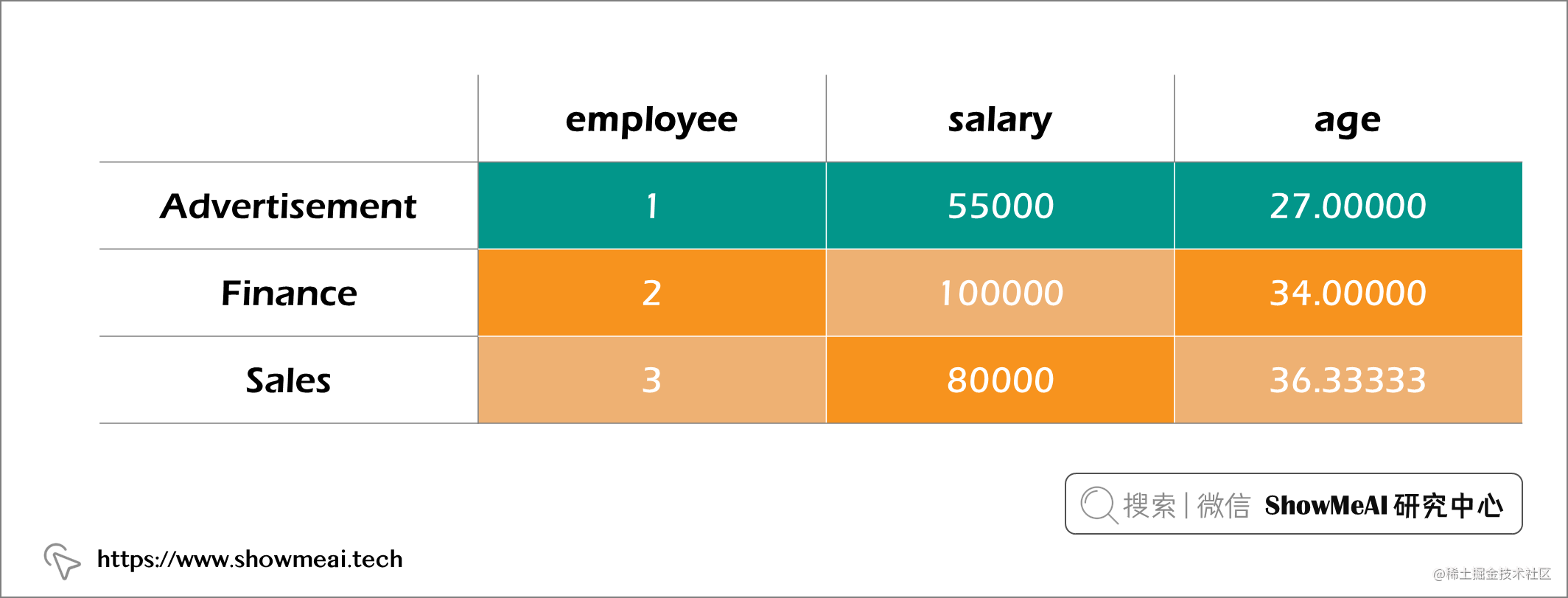``````df.groupby('department').agg({'employee': 'count', 'salary':'max', 'age':'mean'}).reset_index()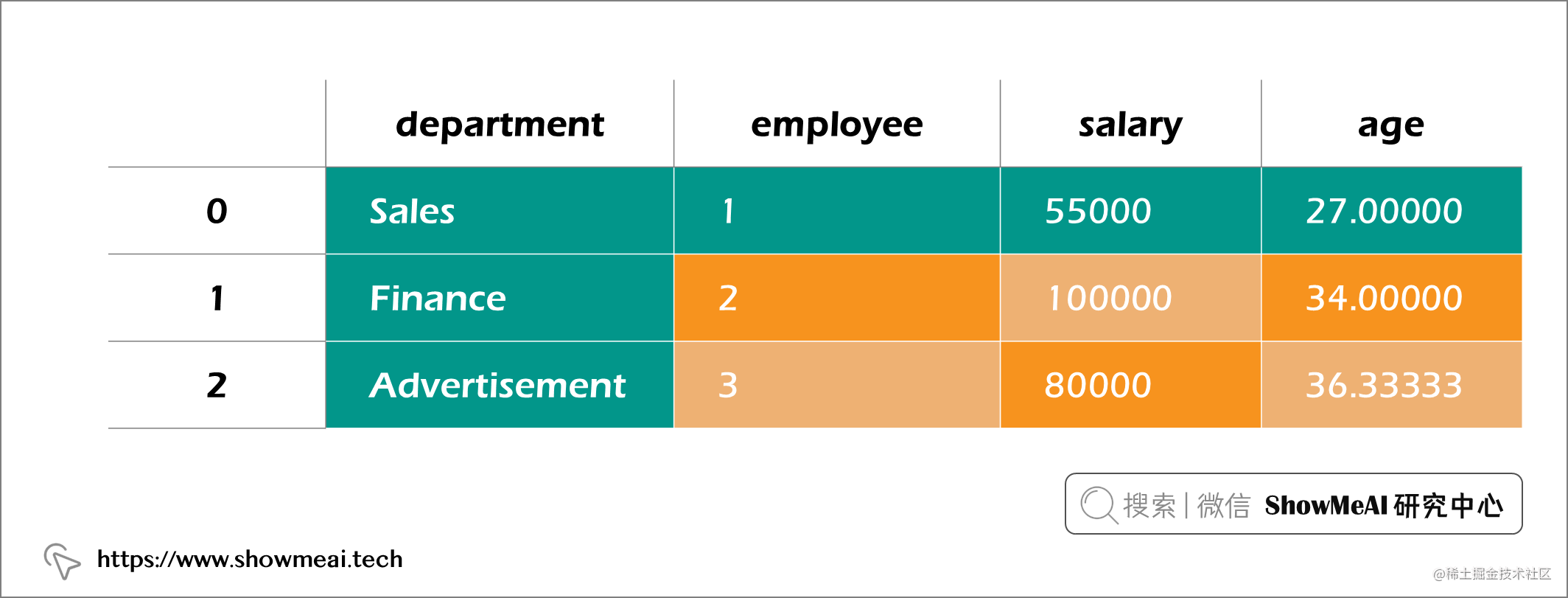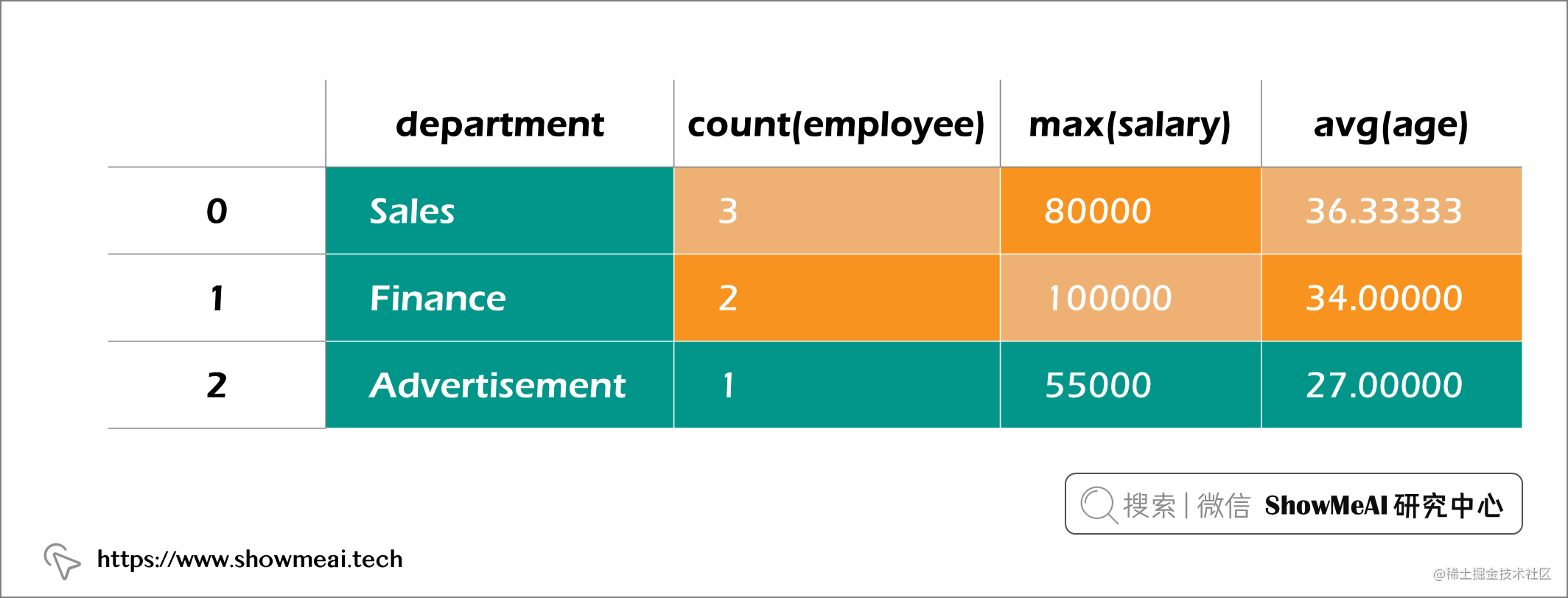``````df.groupBy('department').agg(F.count('employee').alias('employee'), F.max('salary').alias('salary'), F.mean('age').alias('age'))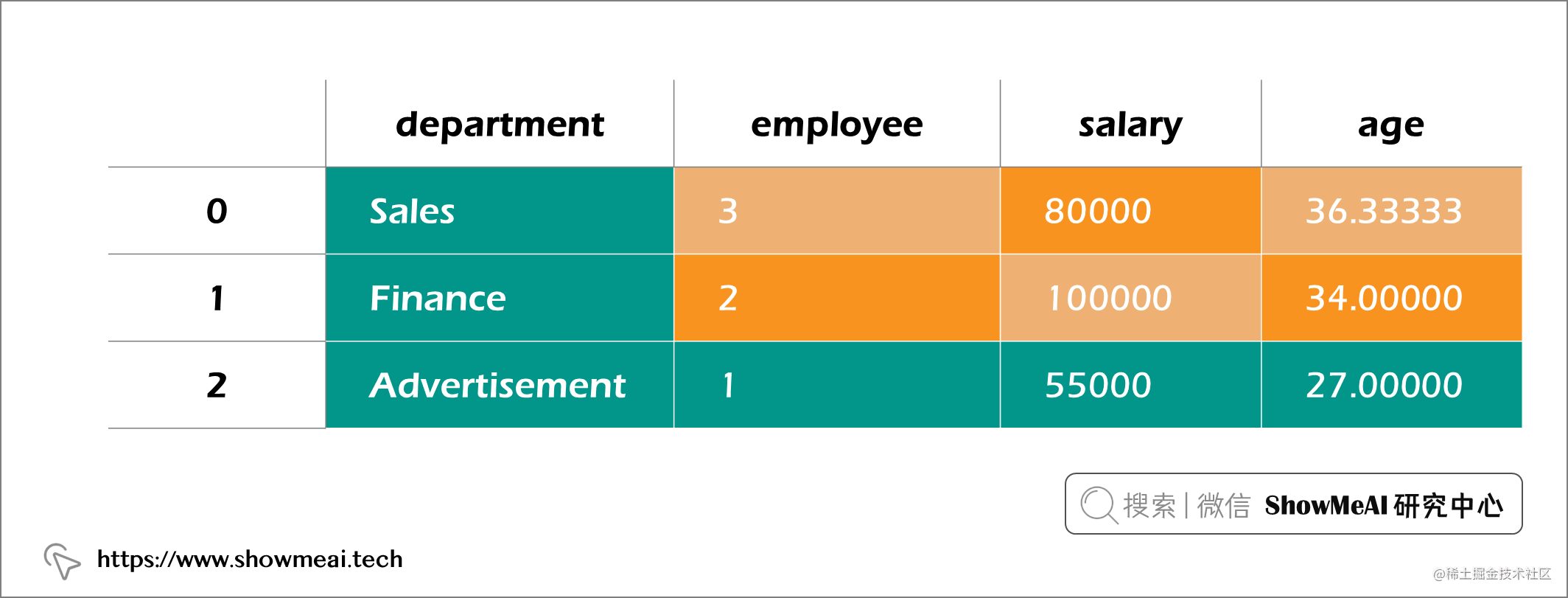# 💡 数据转换

## 💦 Pandas

Pandas 中的语法如下：

``````df['new_salary'] = df['salary'].apply(lambda x: x*1.15 if x<= 60000 else x*1.05)

## 💦 Pyspark

PySpark 中的等价操作下：

``````from pyspark.sql.types import FloatType

df.withColumn('new_salary', F.udf(lambda x: x*1.15 if x<= 60000 else x*1.05, FloatType())('salary'))

⚠️ 请注意， `udf`方法需要明确指定数据类型（在我们的例子中为 FloatType）

# 💡 总结

• 在处理大型数据集时，使用 PySpark 可以为您提供很大的优势，因为它允许并行计算。
• 如果您正在使用的数据集很小，那么使用Pandas会很快和灵活。

# 参考资料